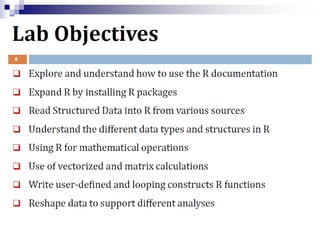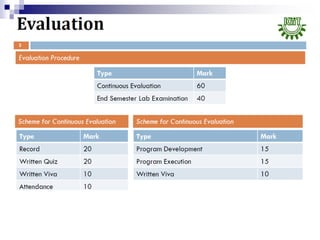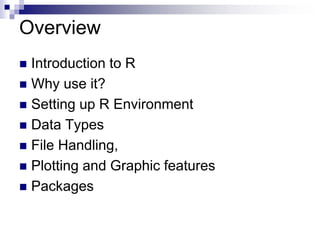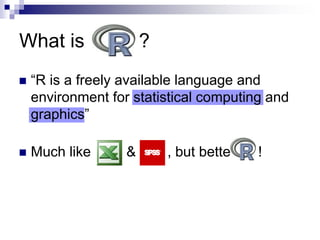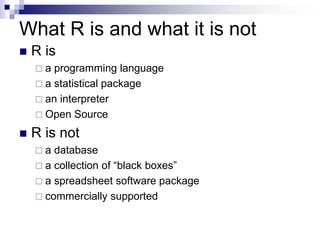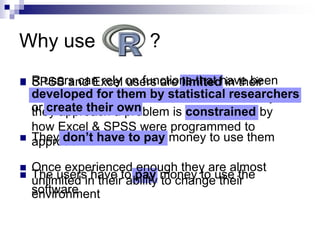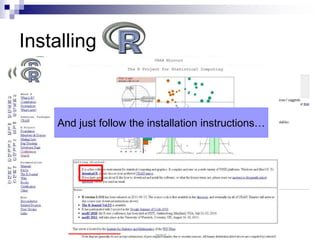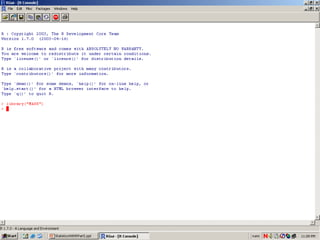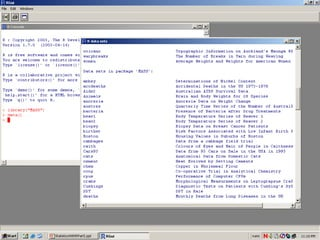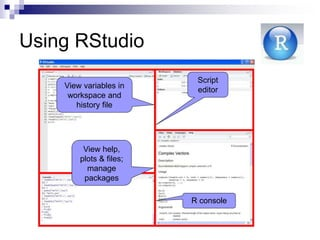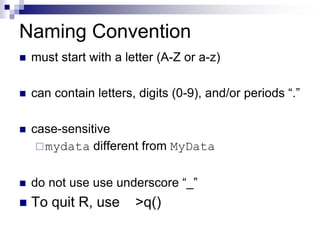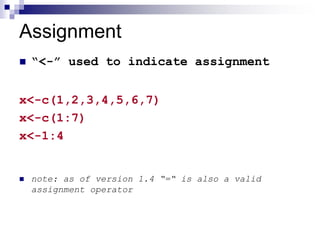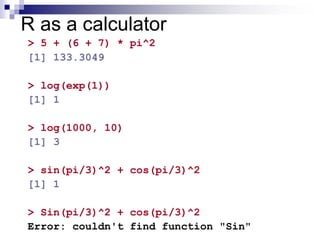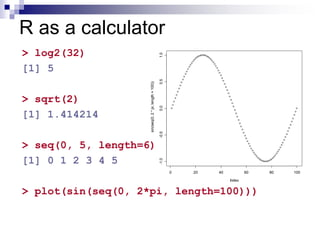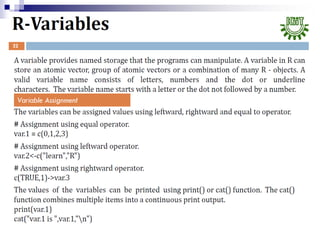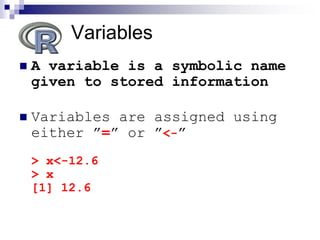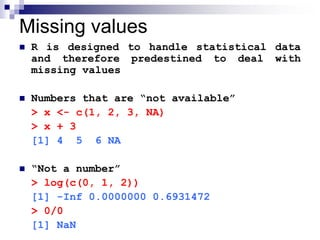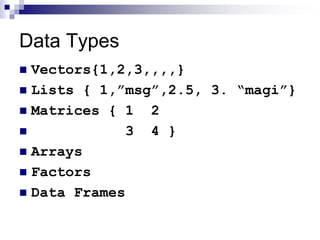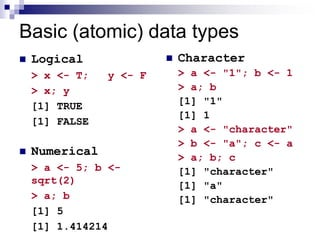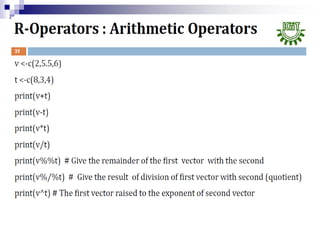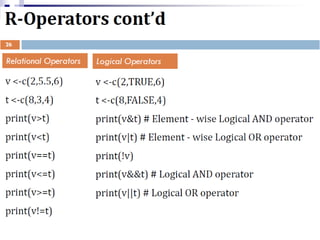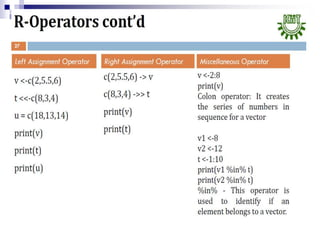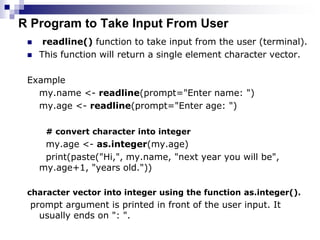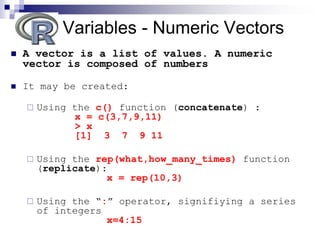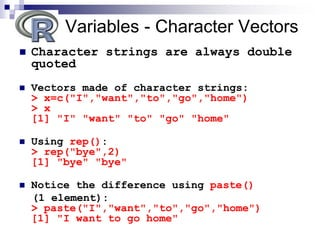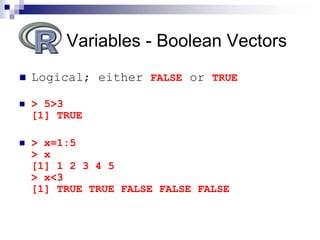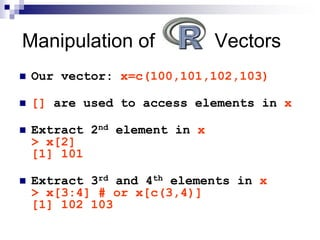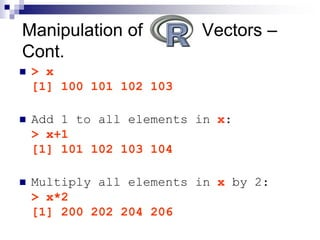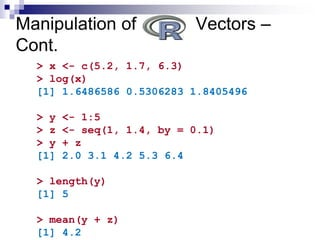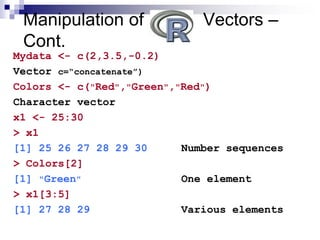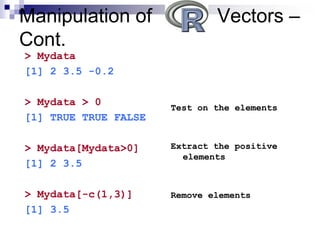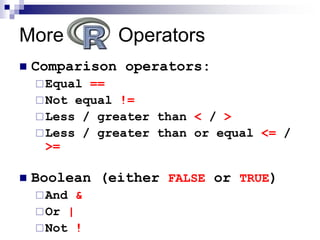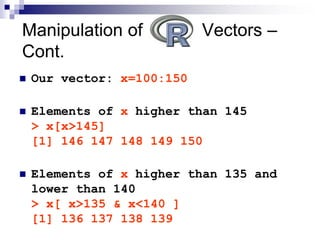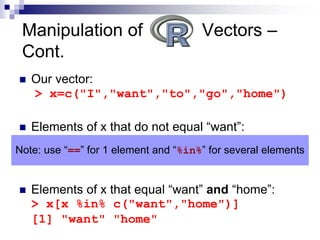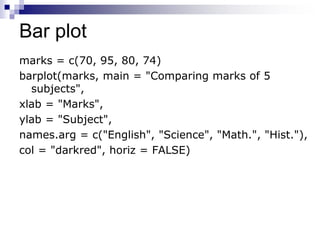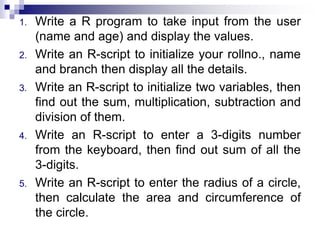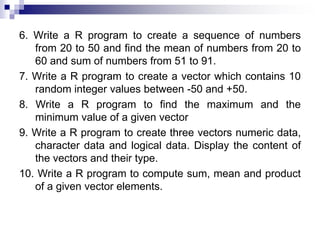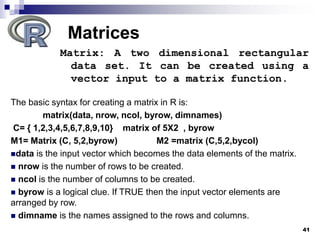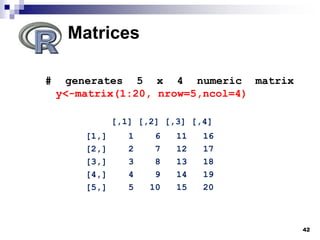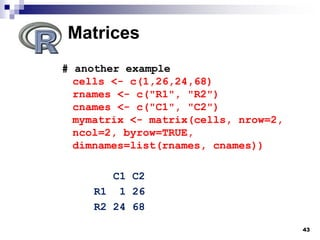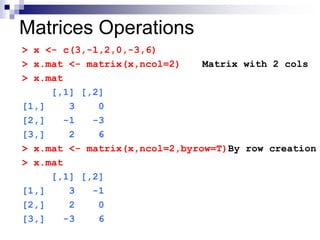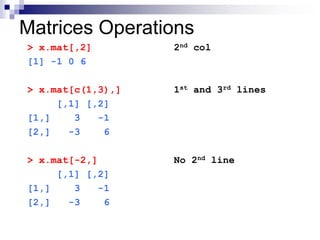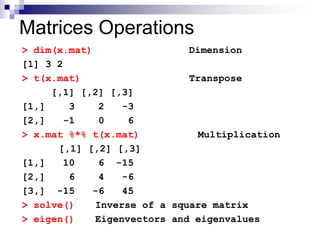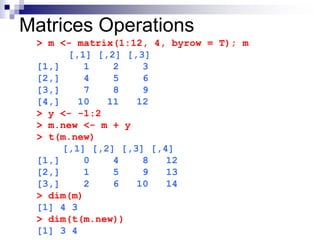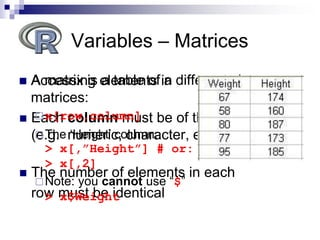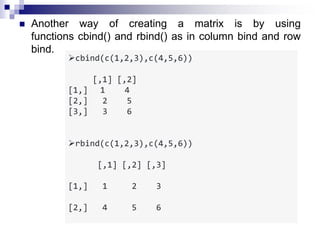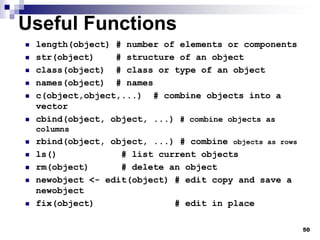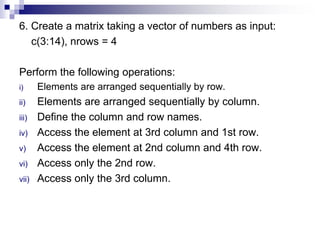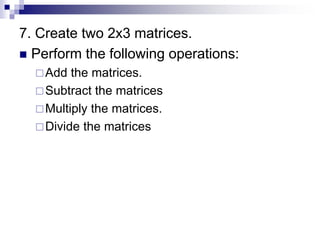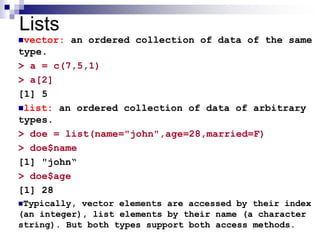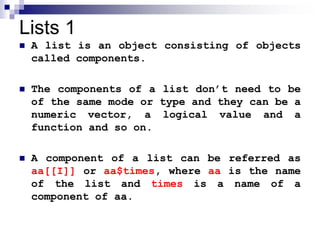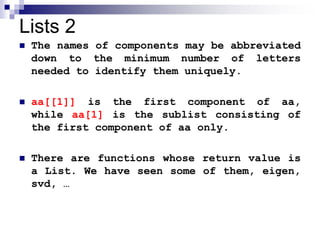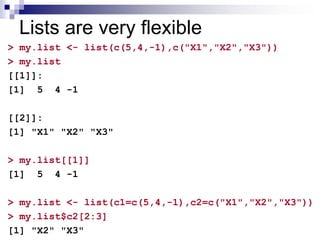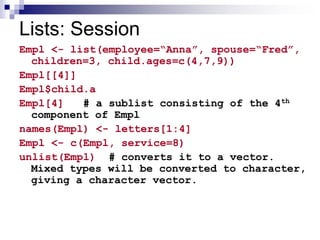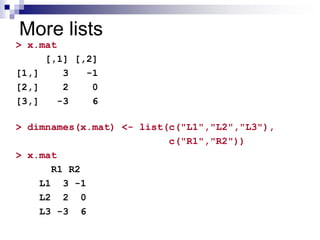1 de 58

### R Programming Intro

1. Programming to School of Computer Engineering, KIIT University 26.6.07
2. Overview  Introduction to R  Why use it?  Setting up R Environment  Data Types  File Handling,  Plotting and Graphic features  Packages
3. What is ?  “R is a freely available language and environment for statistical computing and graphics”  Much like & , but bette !
4. What R is and what it is not  R is  a programming language  a statistical package  an interpreter  Open Source  R is not  a database  a collection of “black boxes”  a spreadsheet software package  commercially supported
5. Why use ?  SPSS and Excel users are limited in their ability to change their environment. The way they approach a problem is constrained by how Excel & SPSS were programmed to approach it  The users have to pay money to use the software  R users can rely on functions that have been developed for them by statistical researchers or create their own  They don’t have to pay money to use them  Once experienced enough they are almost unlimited in their ability to change their environment
6. Installing  Go to R homepage: http://www.r-project.org/ Choose a server And just follow the installation instructions…
7. Getting started  To obtain and install R on your computer  Go to http://cran.r-project.org/mirrors.html to choose a mirror near you  Click on your favorite operating system (Linux, Mac, or Windows)  Download and install the “base”  To install additional packages  Start R on your computer  Choose the appropriate item from the “Packages” menu
8. Installing RStudio  “RStudio is a new integrated development environment (IDE) for R”  Install the “desktop edition” from this link: http://www.rstudio.org/download/
9. Using RStudio Script editor View help, plots & files; manage packages View variables in workspace and history file R console
10. Naming Convention  must start with a letter (A-Z or a-z)  can contain letters, digits (0-9), and/or periods “.”  case-sensitive mydata different from MyData  do not use use underscore “_”  To quit R, use >q()
11. Assignment  “<-” used to indicate assignment x<-c(1,2,3,4,5,6,7) x<-c(1:7) x<-1:4  note: as of version 1.4 “=“ is also a valid assignment operator
12. R as a calculator > 5 + (6 + 7) * pi^2  133.3049 > log(exp(1))  1 > log(1000, 10)  3 > sin(pi/3)^2 + cos(pi/3)^2  1 > Sin(pi/3)^2 + cos(pi/3)^2 Error: couldn't find function "Sin"
13. R as a calculator > log2(32)  5 > sqrt(2)  1.414214 > seq(0, 5, length=6)  0 1 2 3 4 5 > plot(sin(seq(0, 2*pi, length=100))) 0 20 40 60 80 100 -1.0 -0.5 0.0 0.5 1.0 Index sin(seq(0, 2 * pi, length = 100))
14.  A variable is a symbolic name given to stored information  Variables are assigned using either ”=” or ”<-” > x<-12.6 > x  12.6 Variables
15. Missing values  R is designed to handle statistical data and therefore predestined to deal with missing values  Numbers that are “not available” > x <- c(1, 2, 3, NA) > x + 3  4 5 6 NA  “Not a number” > log(c(0, 1, 2))  -Inf 0.0000000 0.6931472 > 0/0  NaN
16. Data Types  Vectors{1,2,3,,,,}  Lists { 1,”msg”,2.5, 3. “magi”}  Matrices { 1 2  3 4 }  Arrays  Factors  Data Frames
17. Basic (atomic) data types  Logical > x <- T; y <- F > x; y  TRUE  FALSE  Numerical > a <- 5; b <- sqrt(2) > a; b  5  1.414214  Character > a <- "1"; b <- 1 > a; b  "1"  1 > a <- "character" > b <- "a"; c <- a > a; b; c  "character"  "a"  "character"
18. R Program to Take Input From User  readline() function to take input from the user (terminal).  This function will return a single element character vector. Example my.name <- readline(prompt="Enter name: ") my.age <- readline(prompt="Enter age: ") # convert character into integer my.age <- as.integer(my.age) print(paste("Hi,", my.name, "next year you will be", my.age+1, "years old.")) character vector into integer using the function as.integer(). prompt argument is printed in front of the user input. It usually ends on ": ".
19.  A vector is a list of values. A numeric vector is composed of numbers  It may be created:  Using the c() function (concatenate) : x = c(3,7,9,11) > x  3 7 9 11  Using the rep(what,how_many_times) function (replicate): x = rep(10,3)  Using the “:” operator, signifiying a series of integers x=4:15 Variables - Numeric Vectors
20.  Character strings are always double quoted  Vectors made of character strings: > x=c("I","want","to","go","home") > x  "I" "want" "to" "go" "home"  Using rep(): > rep("bye",2)  "bye" "bye"  Notice the difference using paste() (1 element): > paste("I","want","to","go","home")  "I want to go home" Variables - Character Vectors
21.  Logical; either FALSE or TRUE  > 5>3  TRUE  > x=1:5 > x  1 2 3 4 5 > x<3  TRUE TRUE FALSE FALSE FALSE Variables - Boolean Vectors
22.  Our vector: x=c(100,101,102,103)  [] are used to access elements in x  Extract 2nd element in x > x  101  Extract 3rd and 4th elements in x > x[3:4] # or x[c(3,4)]  102 103 Manipulation of Vectors
23.  > x  100 101 102 103  Add 1 to all elements in x: > x+1  101 102 103 104  Multiply all elements in x by 2: > x*2  200 202 204 206 Manipulation of Vectors – Cont.
24. Manipulation of Vectors – Cont. > x <- c(5.2, 1.7, 6.3) > log(x)  1.6486586 0.5306283 1.8405496 > y <- 1:5 > z <- seq(1, 1.4, by = 0.1) > y + z  2.0 3.1 4.2 5.3 6.4 > length(y)  5 > mean(y + z)  4.2
25. Mydata <- c(2,3.5,-0.2) Vector c=“concatenate”) Colors <- c("Red","Green","Red") Character vector x1 <- 25:30 > x1  25 26 27 28 29 30 Number sequences > Colors  "Green" One element > x1[3:5]  27 28 29 Various elements Manipulation of Vectors – Cont.
26. Manipulation of Vectors – Cont. Test on the elements Extract the positive elements Remove elements > Mydata  2 3.5 -0.2 > Mydata > 0  TRUE TRUE FALSE > Mydata[Mydata>0]  2 3.5 > Mydata[-c(1,3)]  3.5
27. More Operators  Comparison operators: Equal == Not equal != Less / greater than < / > Less / greater than or equal <= / >=  Boolean (either FALSE or TRUE) And & Or | Not !
28.  Our vector: x=100:150  Elements of x higher than 145 > x[x>145]  146 147 148 149 150  Elements of x higher than 135 and lower than 140 > x[ x>135 & x<140 ]  136 137 138 139 Manipulation of Vectors – Cont.
29.  Our vector: > x=c("I","want","to","go","home")  Elements of x that do not equal “want”: > x[x != "want"]  "I" "to" "go" "home"  Elements of x that equal “want” and “home”: > x[x %in% c("want","home")]  "want" "home" Manipulation of Vectors – Cont. Note: use “==” for 1 element and “%in%” for several elements
30. Bar plot marks = c(70, 95, 80, 74) barplot(marks, main = "Comparing marks of 5 subjects", xlab = "Marks", ylab = "Subject", names.arg = c("English", "Science", "Math.", "Hist."), col = "darkred", horiz = FALSE)
31. 1. Write a R program to take input from the user (name and age) and display the values. 2. Write an R-script to initialize your rollno., name and branch then display all the details. 3. Write an R-script to initialize two variables, then find out the sum, multiplication, subtraction and division of them. 4. Write an R-script to enter a 3-digits number from the keyboard, then find out sum of all the 3-digits. 5. Write an R-script to enter the radius of a circle, then calculate the area and circumference of the circle.
32. 6. Write a R program to create a sequence of numbers from 20 to 50 and find the mean of numbers from 20 to 60 and sum of numbers from 51 to 91. 7. Write a R program to create a vector which contains 10 random integer values between -50 and +50. 8. Write a R program to find the maximum and the minimum value of a given vector 9. Write a R program to create three vectors numeric data, character data and logical data. Display the content of the vectors and their type. 10. Write a R program to compute sum, mean and product of a given vector elements.
33. 41 Matrices Matrix: A two dimensional rectangular data set. It can be created using a vector input to a matrix function. The basic syntax for creating a matrix in R is: matrix(data, nrow, ncol, byrow, dimnames) C= { 1,2,3,4,5,6,7,8,9,10} matrix of 5X2 , byrow M1= Matrix (C, 5,2,byrow) M2 =matrix (C,5,2,bycol) data is the input vector which becomes the data elements of the matrix.  nrow is the number of rows to be created.  ncol is the number of columns to be created.  byrow is a logical clue. If TRUE then the input vector elements are arranged by row.  dimname is the names assigned to the rows and columns.
34. 42 Matrices # generates 5 x 4 numeric matrix y<-matrix(1:20, nrow=5,ncol=4) [,1] [,2] [,3] [,4] [1,] 1 6 11 16 [2,] 2 7 12 17 [3,] 3 8 13 18 [4,] 4 9 14 19 [5,] 5 10 15 20
35. 43 Matrices # another example cells <- c(1,26,24,68) rnames <- c("R1", "R2") cnames <- c("C1", "C2") mymatrix <- matrix(cells, nrow=2, ncol=2, byrow=TRUE, dimnames=list(rnames, cnames)) C1 C2 R1 1 26 R2 24 68
36. Matrices Operations > x <- c(3,-1,2,0,-3,6) > x.mat <- matrix(x,ncol=2) Matrix with 2 cols > x.mat [,1] [,2] [1,] 3 0 [2,] -1 -3 [3,] 2 6 > x.mat <- matrix(x,ncol=2,byrow=T)By row creation > x.mat [,1] [,2] [1,] 3 -1 [2,] 2 0 [3,] -3 6
37. Matrices Operations > x.mat[,2] 2nd col  -1 0 6 > x.mat[c(1,3),] 1st and 3rd lines [,1] [,2] [1,] 3 -1 [2,] -3 6 > x.mat[-2,] No 2nd line [,1] [,2] [1,] 3 -1 [2,] -3 6
38. Matrices Operations > dim(x.mat) Dimension  3 2 > t(x.mat) Transpose [,1] [,2] [,3] [1,] 3 2 -3 [2,] -1 0 6 > x.mat %*% t(x.mat) Multiplication [,1] [,2] [,3] [1,] 10 6 -15 [2,] 6 4 -6 [3,] -15 -6 45 > solve() Inverse of a square matrix > eigen() Eigenvectors and eigenvalues
39. Matrices Operations > m <- matrix(1:12, 4, byrow = T); m [,1] [,2] [,3] [1,] 1 2 3 [2,] 4 5 6 [3,] 7 8 9 [4,] 10 11 12 > y <- -1:2 > m.new <- m + y > t(m.new) [,1] [,2] [,3] [,4] [1,] 0 4 8 12 [2,] 1 5 9 13 [3,] 2 6 10 14 > dim(m)  4 3 > dim(t(m.new))  3 4
40.  A matrix is a table of a different class  Each column must be of the same class (e.g. numeric, character, etc.)  The number of elements in each row must be identical Variables – Matrices  Accessing elements in matrices: x[row,column] The ‘Height’ column: > x[,”Height”] # or: > x[,2] Note: you cannot use “\$” > x\$Weight
41.  Another way of creating a matrix is by using functions cbind() and rbind() as in column bind and row bind. cbind(c(1,2,3),c(4,5,6)) [,1] [,2] [1,] 1 4 [2,] 2 5 [3,] 3 6 rbind(c(1,2,3),c(4,5,6)) [,1] [,2] [,3] [1,] 1 2 3 [2,] 4 5 6
42. 50 Useful Functions  length(object) # number of elements or components  str(object) # structure of an object  class(object) # class or type of an object  names(object) # names  c(object,object,...) # combine objects into a vector  cbind(object, object, ...) # combine objects as columns  rbind(object, object, ...) # combine objects as rows  ls() # list current objects  rm(object) # delete an object  newobject <- edit(object) # edit copy and save a newobject  fix(object) # edit in place
43. 6. Create a matrix taking a vector of numbers as input: c(3:14), nrows = 4 Perform the following operations: i) Elements are arranged sequentially by row. ii) Elements are arranged sequentially by column. iii) Define the column and row names. iv) Access the element at 3rd column and 1st row. v) Access the element at 2nd column and 4th row. vi) Access only the 2nd row. vii) Access only the 3rd column.
44. 7. Create two 2x3 matrices.  Perform the following operations: Add the matrices. Subtract the matrices Multiply the matrices. Divide the matrices
45. Lists vector: an ordered collection of data of the same type. > a = c(7,5,1) > a  5 list: an ordered collection of data of arbitrary types. > doe = list(name="john",age=28,married=F) > doe\$name  "john“ > doe\$age  28 Typically, vector elements are accessed by their index (an integer), list elements by their name (a character string). But both types support both access methods.
46. Lists 1  A list is an object consisting of objects called components.  The components of a list don’t need to be of the same mode or type and they can be a numeric vector, a logical value and a function and so on.  A component of a list can be referred as aa[[I]] or aa\$times, where aa is the name of the list and times is a name of a component of aa.
47. Lists 2  The names of components may be abbreviated down to the minimum number of letters needed to identify them uniquely.  aa[] is the first component of aa, while aa is the sublist consisting of the first component of aa only.  There are functions whose return value is a List. We have seen some of them, eigen, svd, …
48. Lists are very flexible > my.list <- list(c(5,4,-1),c("X1","X2","X3")) > my.list []:  5 4 -1 []:  "X1" "X2" "X3" > my.list[]  5 4 -1 > my.list <- list(c1=c(5,4,-1),c2=c("X1","X2","X3")) > my.list\$c2[2:3]  "X2" "X3"
49. Lists: Session Empl <- list(employee=“Anna”, spouse=“Fred”, children=3, child.ages=c(4,7,9)) Empl[] Empl\$child.a Empl # a sublist consisting of the 4th component of Empl names(Empl) <- letters[1:4] Empl <- c(Empl, service=8) unlist(Empl) # converts it to a vector. Mixed types will be converted to character, giving a character vector.
50. More lists > x.mat [,1] [,2] [1,] 3 -1 [2,] 2 0 [3,] -3 6 > dimnames(x.mat) <- list(c("L1","L2","L3"), c("R1","R2")) > x.mat R1 R2 L1 3 -1 L2 2 0 L3 -3 6